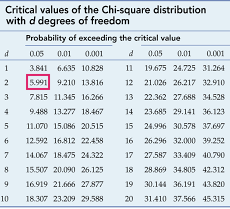# Python – Pearson’s Chi-Square Test

• Difficulty Level : Basic
• Last Updated : 23 Jun, 2020

The Pearson’s Chi-Square statistical hypothesis is a test for independence between categorical variables. In this article, we will perform the test using a mathematical approach and then using Python’s SciPy module.
First, let us see the mathematical approach :

The Contingency Table :
A Contingency table (also called crosstab) is used in statistics to summarise the relationship between several categorical variables. Here, we take a table that shows the number of men and women buying different types of pets.

The aim of the test is to conclude whether the two variables( gender and choice of pet ) are related to each other.

Null hypothesis:
We start by defining the null hypothesis (H0) which states that there is no relation between the variables. An alternate hypothesis would state that there is a significant relation between the two.

We can verify the hypothesis by these methods:

• Using p-value:

We define a significance factor to determine whether the relation between the variables is of considerable significance. Generally a significance factor or alpha value of 0.05 is chosen. This alpha value denotes the probability of erroneously rejecting H0 when it is true. A lower alpha value is chosen in cases where we expect more precision. If the p-value for the test comes out to be strictly greater than the alpha value, then H0 holds true.

• Using chi-square value:

If our calculated value of chi-square is less or equal to the tabular(also called critical) value of chi-square, then H0 holds true.

Expected Values Table :

Next, we prepare a similar table of calculated(or expected) values. To do this we need to calculate each item in the new table as :The expected values table :

Chi-Square Table :

We prepare this table by calculating for each item the following:The chi-square table:

From this table, we obtain the total of the last column, which gives us the calculated value of chi-square.  Hence the calculated value of chi-square is 4.542228269825232

Now, we need to find the critical value of chi-square. We can obtain this from a table. To use this table, we need to know the degrees of freedom for the dataset.  The degrees of freedom is defined as : (no. of rows – 1) * (no. of columns – 1).
Hence, the degrees of freedom is (2-1) * (3-1) = 2

Now, let us look at the table and find the value corresponding to 2 degrees of freedom and 0.05 significance factor :The tabular or critical value of chi-square here is  5.991

Hence,Therefore, H0 is accepted, that is, the variables do not have a significant relation.

Next, let us see how to perform the test in Python.

Performing the test using Python (scipy.stats) :

SciPy is an Open Source Python library, which is used in mathematics, engineering, scientific and technical computing.

Installation:

pip install scipy


The chi2_contingency() function of scipy.stats module takes as input, the contingency table in 2d array format. It returns a tuple containing test statistics, the p-value, degrees of freedom and expected table(the one we created from the calculated values) in that order.

Hence, we need to compare the obtained p-value with alpha value of 0.05.

 from scipy.stats import chi2_contingency  # defining the tabledata = [[207, 282, 241], [234, 242, 232]]stat, p, dof, expected = chi2_contingency(data)  # interpret p-valuealpha = 0.05print("p value is " + str(p))if p <= alpha:    print('Dependent (reject H0)')else:    print('Independent (H0 holds true)')

Output :

p value is 0.1031971404730939
Independent (H0 holds true)


Since,

p-value > alpha

Therefore, we accept H0, that is, the variables do not have a significant relation.

My Personal Notes arrow_drop_up